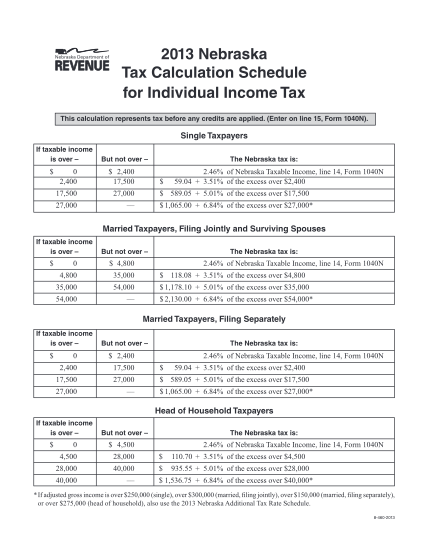2013 Nebraska Tax Calculation Schedule for Individual Income Tax This calculation represents tax...2013 Nebraska Tax Calculation Schedule for Individual Income Tax This calculation represents tax...2013 Nebraska Tax Calculation Schedule for Individual Income Tax This calculation represents tax...2013 Nebraska Tax Calculation Schedule for Individual Income Tax This calculation represents tax...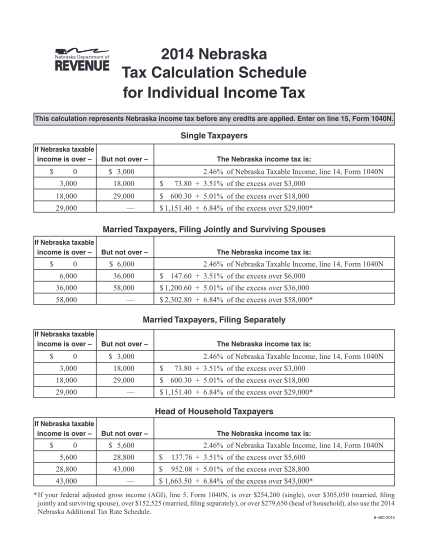2014 Nebraska Tax Calculation Schedule for Individual Income Tax This calculation represents...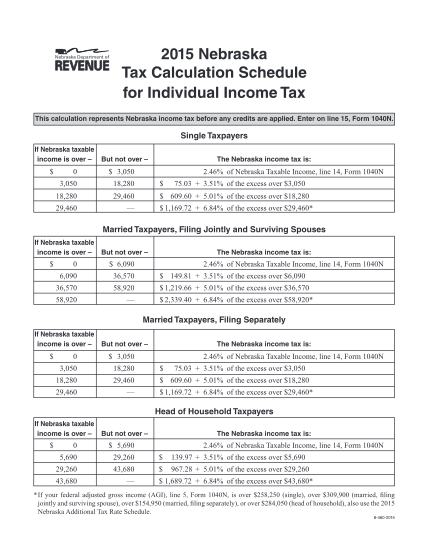2015 Nebraska Tax Calculation Schedule for Individual Income Tax This calculation represents...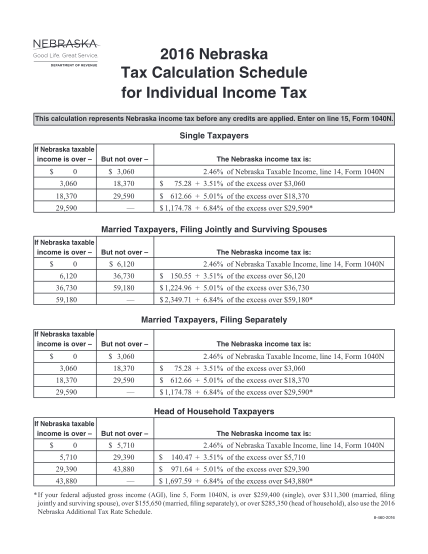2016 Nebraska Tax Calculation Schedule for Individual Income Tax This calculation represents...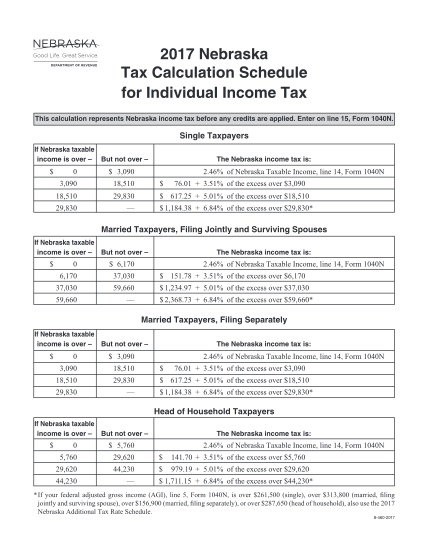2017 Nebraska Tax Calculation Schedule for Individual Income Tax This calculation represents...2017 Nebraska Tax Calculation Schedule for Individual Income Tax This calculation represents...2017 Nebraska Tax Calculation Schedule for Individual Income Tax This calculation represents...